# Check for balancing, use lowest set of whole number coefficients, and what type of reaction is....

Check for balancing, use lowest set of whole number coefficients, and what type of reaction is.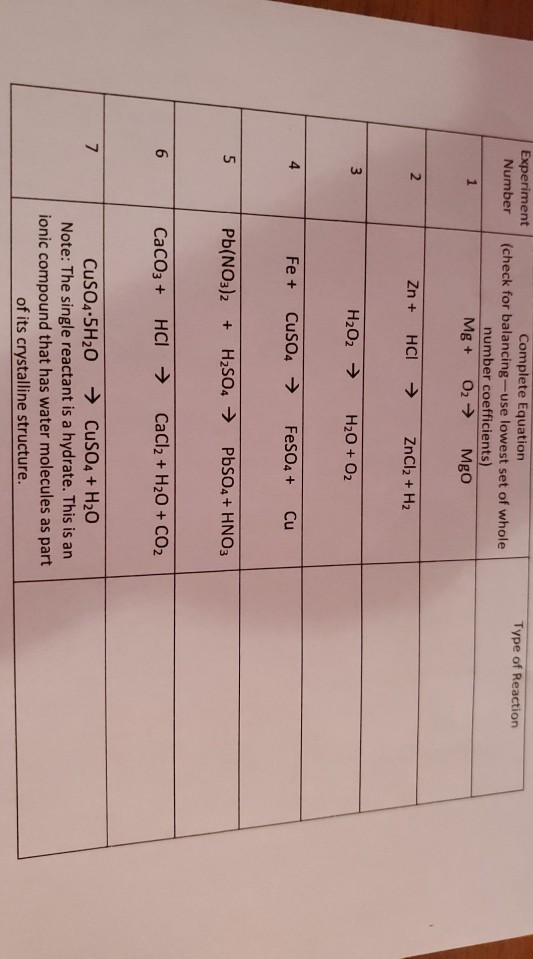Experiment Number Type of Reaction Complete Equation (check for balancing-use lowest set of whole number coefficients) Mg + 02 → Mgo 1 2. Zn + HCI ZnCl2 + H2 با H2O2 → H2O + O2 4 Fe + CuSO4 → FeSO4 + Cu 5 Pb(NO3)2 + H2SO4 → PbSO4 + HNO3 6 CaCO3 + HCl → CaCl2 + H2O + CO2 7 CuSO4.5H2O → CuSO4 + H2O Note: The single reactant is a hydrate. This is an ionic compound that has water molecules as part of its crystalline structure.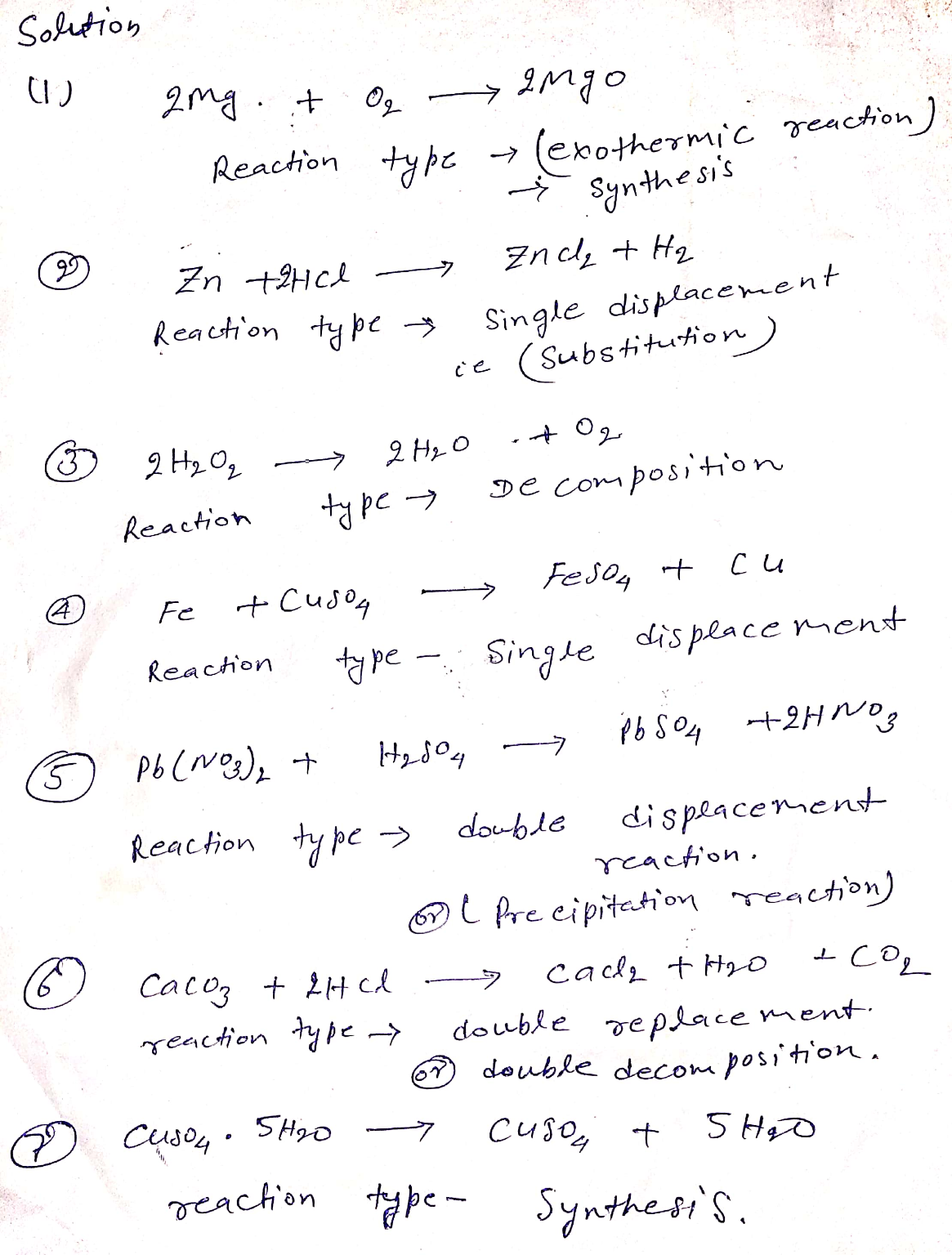##### Add Answer of: Check for balancing, use lowest set of whole number coefficients, and what type of reaction is....
Similar Homework Help Questions
• ### Complete equation, check for balancing, use lowest set of whole number coefficients. 4. Complete the Post...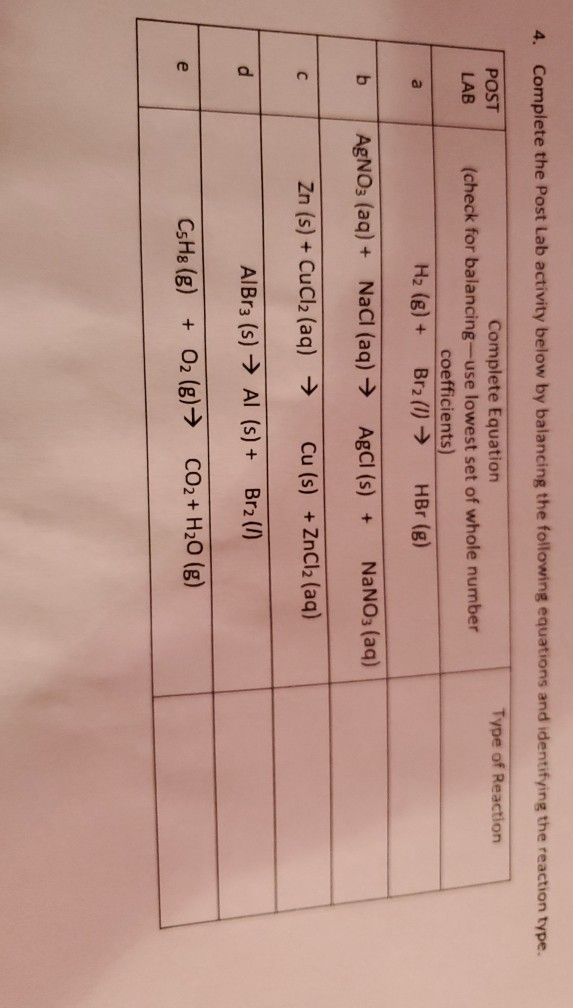Complete equation, check for balancing, use lowest set of whole number coefficients. 4. Complete the Post Lab activity below by balancing the following equations and identifying the reaction type. POST Type of Reaction LAB Complete Equation (check for balancing-use lowest set of whole number coefficients) Hz (g) + Br2 (1) ► HBr (g) a b AgNO3 (aq) + NaCl (aq) → AgCl (s) + NaNO3(aq) с Zn (s) + CuCl2 (aq) → Cu (s) + ZnCl2 (aq) d AlBr3 (s)...

• ### CHEM 110 Online Lab Instructions for Exp. 7 Types of Chemical Reactions 1. Read sections 8.1...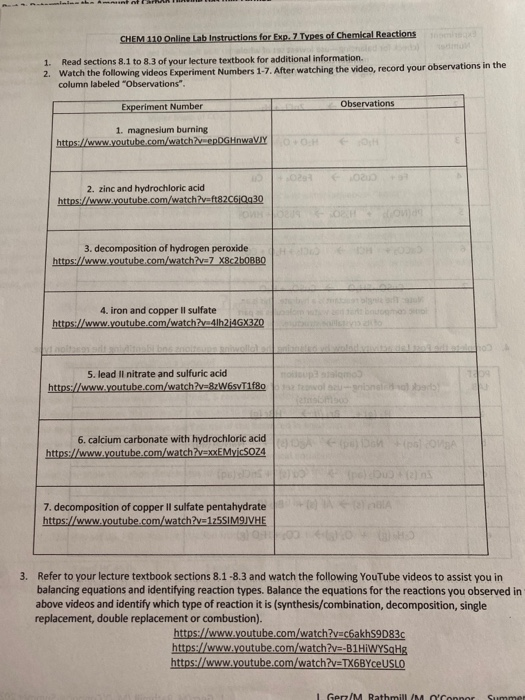CHEM 110 Online Lab Instructions for Exp. 7 Types of Chemical Reactions 1. Read sections 8.1 to 8.3 of your lecture textbook for additional information. 2. Watch the following videos Experiment Numbers 1-7. After watching the video, record your observations in the column labeled "Observations". Experiment Number Observations 1. magnesium burning https://www.youtube.com/watchrepDGHnWaVIY 2. zinc and hydrochloric acid https://www.youtube.com/watch?vaft82C6Qg30 3. decomposition of hydrogen peroxide https://www.youtube.com/watch?v=7 X8c2bOBBO 4. iron and copper il sulfate https://www.youtube.com/watch?v4lh24GX320 5. lead Il nitrate and sulfuric acid https://www.youtube.com/watch?v=8W65711f8o...

• ### check for balancing Zn +     HCl gives      ZnCl2 +H2 and what type of reaction is?

check for balancing Zn +     HCl gives      ZnCl2 +H2 and what type of reaction is?

• ### Balance the following equations. (Use the lowest possible whole-number coefficients. These may be zero.) (a) Cr202-(aq)...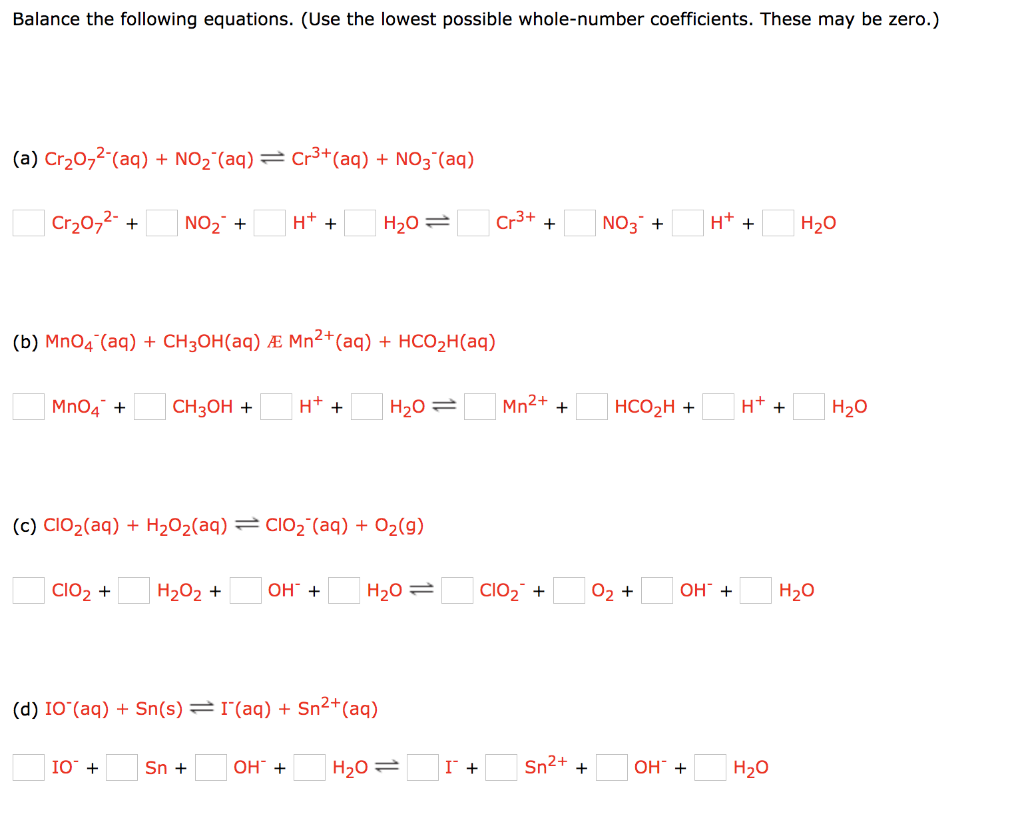Balance the following equations. (Use the lowest possible whole-number coefficients. These may be zero.) (a) Cr202-(aq) + NO2 (aq) = Cr3+ (aq) + NO3"(aq) Cr20,2- + NO3 + H+ + H2O= Cr3+ + NO3 + H+ + H20 (b) Mn04 (aq) + CH3OH(aq) Æ Mn2+ (aq) + HCO2H(aq) MnO4 + CH3OH + H+ + H2O= Mn2+ + HCO2H + H+ + H2O (c) ClO2(aq) + H2O2(aq) = C102 (aq) + O2(9) C102 + H2O2 + OH + H20 = ClO2...

• ### Balance the following redox reaction if it occurs in basic solution. Provide whole-number coefficients in the...

Balance the following redox reaction if it occurs in basic solution. Provide whole-number coefficients in the areas provided. H2O2(l) + ClO2(aq) → ClO2⁻(aq) + O2(g) H2O2 ClO2 ClO2⁻ O2 OH- H2O

• ### Question 1 1 pts Balance the following reaction with the lowest whole number coefficients: CzH6(g) + O2(g) → CO2(g)...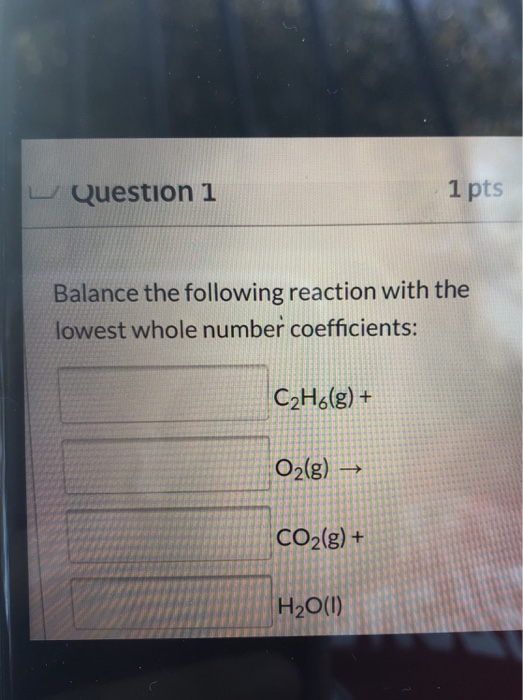Question 1 1 pts Balance the following reaction with the lowest whole number coefficients: CzH6(g) + O2(g) → CO2(g) + H2O(1)

• ### need help balancing the 1st equation then name what type of reaction is occurring for each equation A HNO3(aq) + Cu(...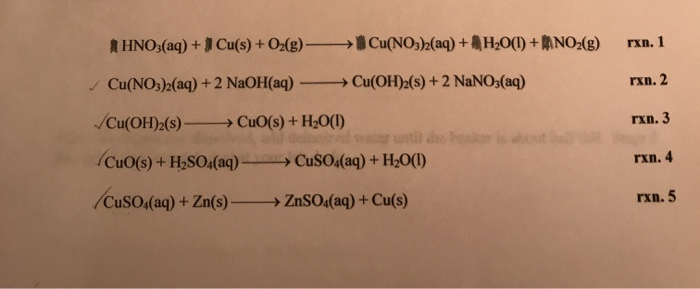need help balancing the 1st equation then name what type of reaction is occurring for each equation A HNO3(aq) + Cu(s) + O2(g) - Cu(NO3)2(aq) + 4H2O(l) +BANO2(g) Cu(NO3)2(aq) + 2 NaOH(aq) - Cu(OH)2(s) +2 NaNO3(aq) rxn. 2 Cu(OH)2(s) - CuO(s) + H2O(l) rxn. 3 rxn. 4 Cuo(s) + H2SO4(aq) CuSO.(aq) + Zn(s) — CuSO4(aq) + H2O(l) + ZnSO4(aq) + Cu(s) rxn. 5

• ### Balance the following equations. (Use the lowest possible whole-number coefficients. These may be zero.) (a) NO3-...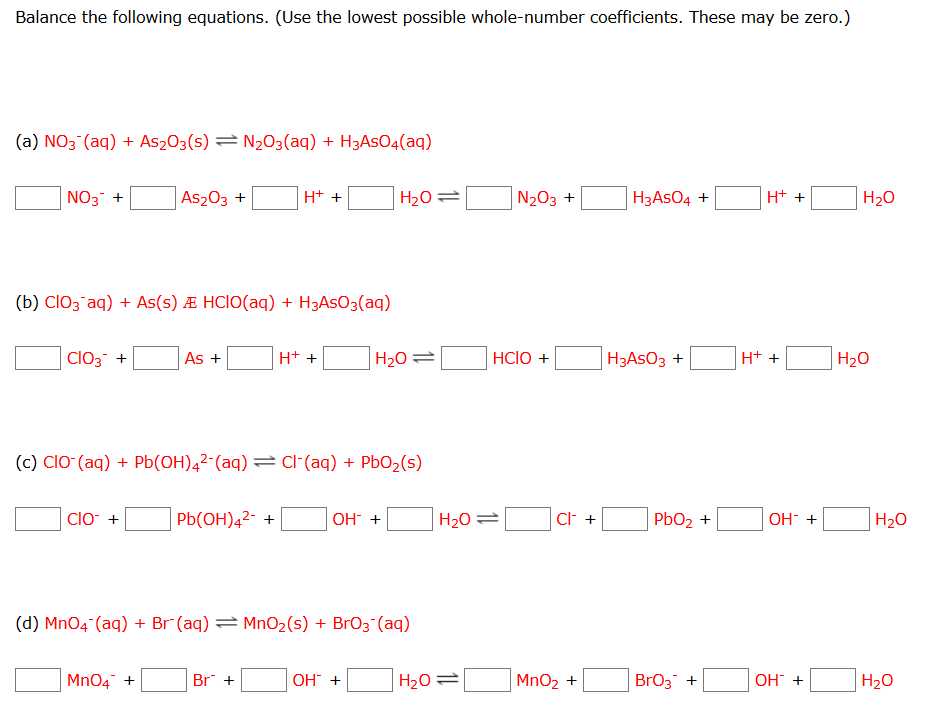Balance the following equations. (Use the lowest possible whole-number coefficients. These may be zero.) (a) NO3- (aq) + As2O3(s) = N2O3(aq) + H3ASO4(aq) N03* + As2O3 + H+ + O H20=O N203+ H3A504 + H+ + H20 (b) Clozaq) + As(s) Æ HClO(aq) + H3AsO3(aq) DC103+ As + OH+ + H2O=OHCO + H2A503 + H+ + OH20 (c) CIO-(aq) + Pb(OH)42-(aq) = Cl(aq) + PbO2(s) D clo + Pb(OH)42- + D OH + H2O=D C + PbO2 + OH +...

• ### Balance the following equation with the lowest set of whole number coefficients in a basic aqueous...Balance the following equation with the lowest set of whole number coefficients in a basic aqueous solution. Bra() +OH(aq) - BrO3(aq) + Br" (aq) + H20 What is the coefficient of H20 in the balanced equation? O A 1 O B.2 OC. 3 D.A E. 6 Show transcribed image text

• ### Balance the following equations. (Use the lowest possible whole-number coefficients. These may be zero.) Balance the...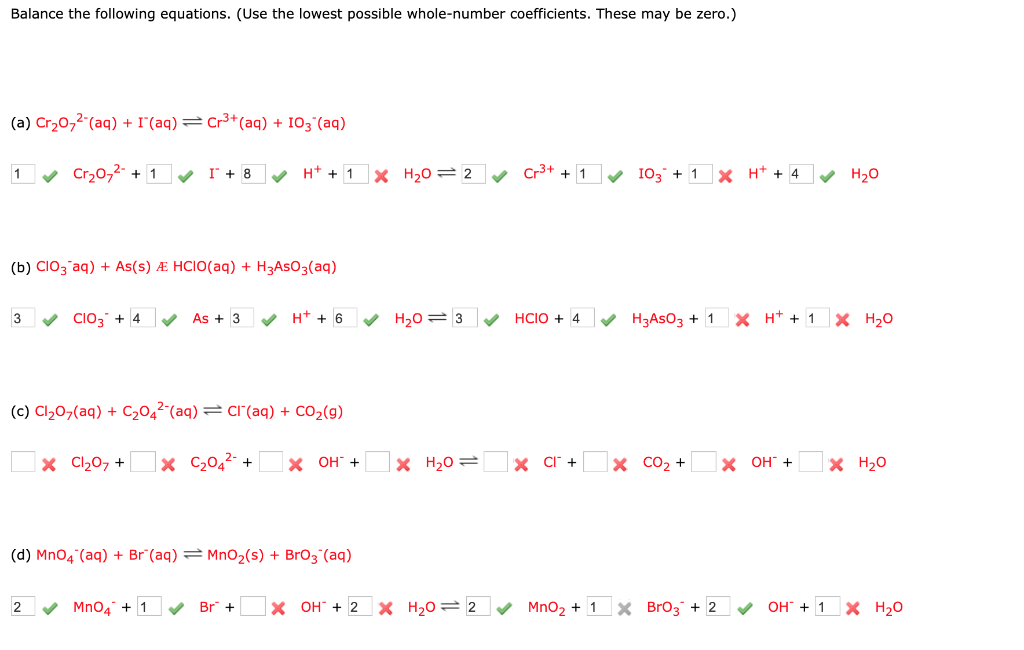Balance the following equations. (Use the lowest possible whole-number coefficients. These may be zero.) Balance the following equations. (Use the lowest possible whole-number coefficients. These may be zero.) (a) Cr2022(aq) + I (aq) = Cr3+ (aq) + 103 (aq) 1 Cr2O72- + 1 1 + 8 H+ + 1 X H20 = 2 Cr3+ + 1 103 + 1 X H+ + 4 H2O (b) CIO3-aq) + As(s) Æ HCIO(aq) + H3A5O3(aq) 3 CIO3 + 4 As + 3 H...

Free Homework App